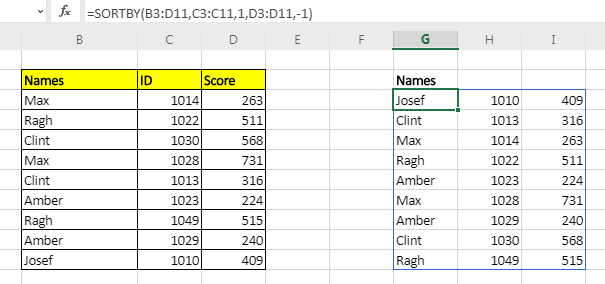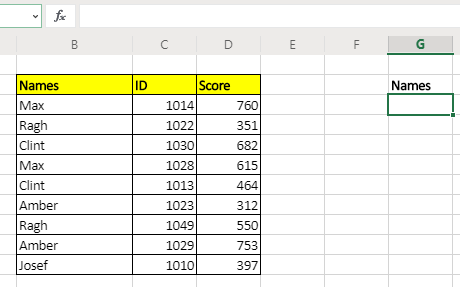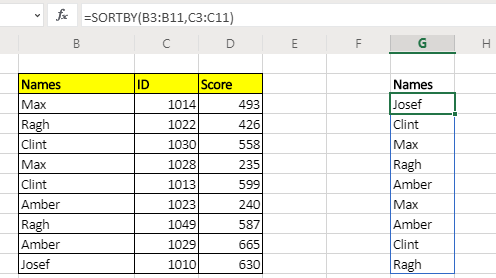How to Use Excel SORTBY FunctionThe SORTBY Function of Excel can return a sorted array by another independent array. You can add levels of sorting. In other words you can sort arrays by more than one column. This function is available to Excel 365 and 2019 users.
Unlike The SORT function, the sorting array does not need to be part of the array and you can add multiple levels of sorting too with SORTBY function.

Syntax of The SORTBY Function

=SORTBY(array,sorting_array1,[order],...)

Array: This is the array that you want to sort.

Sorting_array1: This is the array by which you want to sort the array. The dimension of this array should be compatible with the array.

[order]: Optional. Set it to -1 if you want the order to be descending. By default it is ascending(1).

Let’s see an example to make things clear.

…: This is a continuous function and you can give multiple sorting_array and order. First sorting array is essential. Rest are optional.

Let's have some examples to see how this sorting function works.

Example: Sort range by another range using SORTBY function

Here I have sample data of participants with their IDs and scores. I want to get the names sorted by their IDs using the SORTBY function.Apply above generic formula here to get the ascendingly sorted names by ID column.

=SORTBY(B3:B11,C3:C11)

This will return just the names by ascendingly sorted ID.If you want to get whole table than just give reference of whole table

=SORTBY(B3:D11,C3:C11)

If you want the range to be sorted descendingly by ID column then the formula will be:

=SORTBY(B3:D11,C3:C11,-1)

Example: Sort Array by More Than One Column
In the above examples we sorted array by just one column. But what if we want to do multi level sorting? Well, this function allows you to do multi level sorting.
Let's say you want to first sort the above table ascendingly by Id and then sort the array descendingly by the score. Then the formula will be:

=SORTBY(B3:D11,C3:C11,1,D3:D11,-1)

How does it work?

This exactly works as the sorting of data menu works. The excel hides the internal working of the function.

The benefit of it is of course that you can use it with other functions. You can use the resulting array as input to another function.

Note: This function is not available in Excel 2016 or older. This function is available to excel 2019 and Excel 365. But you can of course use other techniques to sort arrays in excel 2016.

Related Articles:

The SORT Function in Excel | The SORT function  returns the sorted array by the given column number in the array. It also works on horizontal data.

Sort numbers using Excel SMALL Function | To sort numbers using formula in Excel 2016 and older, you can use the SMALL function with other helping functions.

Sort Numeric Values with Excel RANK Function | To sort the numeric values we can use the Rank function. The formula is

Excel Formula to Sort Text | To sort text values using formula in excel we simply use the COUNTIF function. Here is the formula

Popular Articles:

50 Excel Shortcuts to Increase Your Productivity | Get faster at your task. These 50 shortcuts will make you work even faster on Excel.

How to use the VLOOKUP Function in Excel | This is one of the most used and popular functions of excel that is used to lookup value from different ranges and sheets.

How to use the COUNTIF function in Excel 2016 | Count values with conditions using this amazing function. You don't need to filter your data to count specific values. Countif function is essential to prepare your dashboard.

How to use the SUMIF Function in Excel | This is another dashboard essential function. This helps you sum up values on specific conditions.

Terms and Conditions of use

The applications/code on this site are distributed as is and without warranties or liability. In no event shall the owner of the copyrights, or the authors of the applications/code be liable for any loss of profit, any problems or any damage resulting from the use or evaluation of the applications/code.